### Monotone and FCS Predictive Mean Matching Methods

The predictive mean matching method is also an imputation method available for continuous variables. It is similar to the regression method except that for each missing value, it imputes a value randomly from a set of observed values whose predicted values are closest to the predicted value for the missing value from the simulated regression model (Heitjan and Little, 1991; Schenker and Taylor, 1996).

Following the description of the model in the section Monotone and FCS Regression Methods, the following steps are used to generate imputed values:

1. New parameters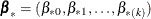and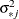are drawn from the posterior predictive distribution of the parameters. That is, they are simulated from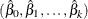,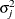, and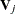. The variance is drawn as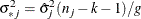where g is a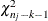random variate and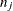is the number of nonmissing observations for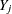. The regression coefficients are drawn as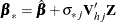where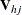is the upper triangular matrix in the Cholesky decomposition,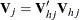, andis a vector of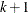independent random normal variates.

2. For each missing value, a predicted value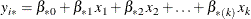is computed with the covariate values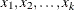.

3. A set of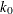observations whose corresponding predicted values are closest to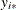is generated. You can specifywith the K= option.

4. The missing value is then replaced by a value drawn randomly from theseobserved values.

The predictive mean matching method requires the number of closest observations to be specified. A smallertends to increase the correlation among the multiple imputations for the missing observation and results in a higher variability of point estimators in repeated sampling. On the other hand, a largertends to lessen the effect from the imputation model and results in biased estimators (Schenker and Taylor, 1996, p. 430).

The predictive mean matching method ensures that imputed values are plausible; it might be more appropriate than the regression method if the normality assumption is violated (Horton and Lipsitz, 2001, p. 246).